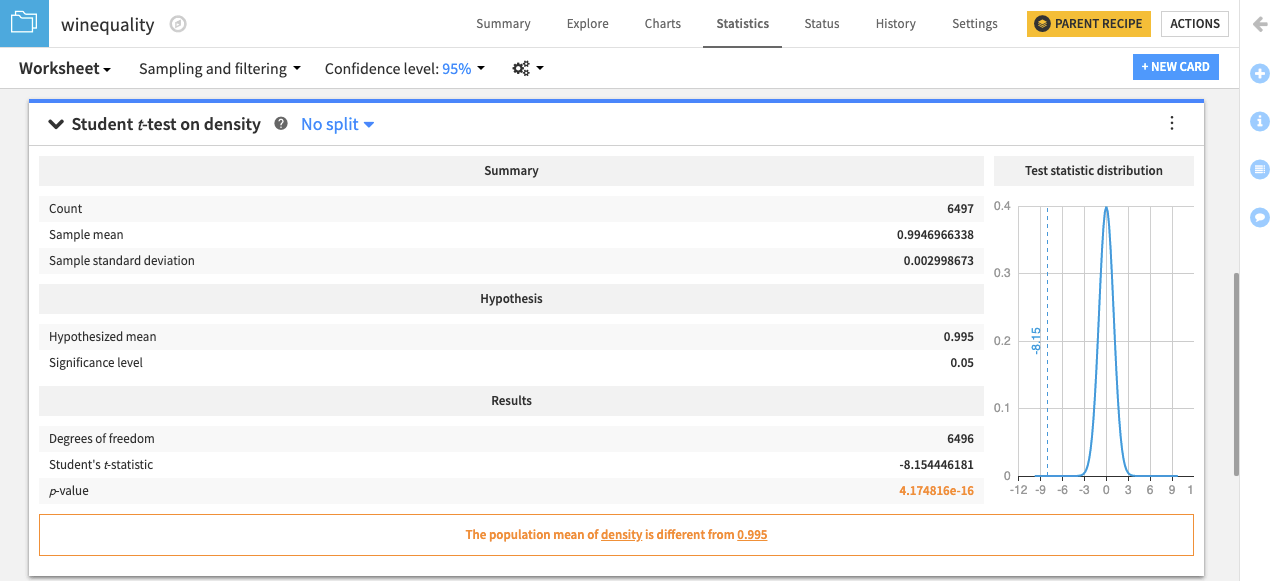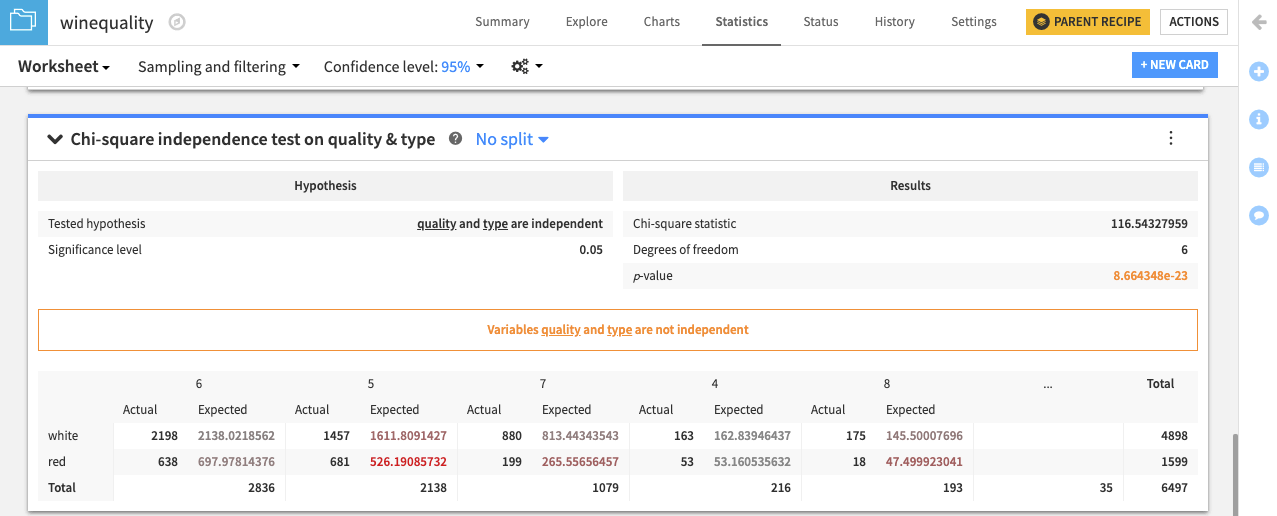# Hands-On: Perform Statistical Tests¶

Note

This lesson is a continuation of the Interactive Visual Statistics hands-on tutorial.

We can make data-driven conclusions from our winequality dataset using Dataiku’s built-in statistical tests. These statistical tests are a form of inferential statistics that use a sample to make predictions about a population. In other words, these tests allow you to test hypotheses about a population using a sample.

This tutorial will walk you through a one-sample test and a categorical test. If you want to find out more about all of our built-in tests, visit our article on Statistical Tests.

## One-sample Student t-test¶

One-sample tests compare the location parameters or distribution of a population to a hypothesis using one sample. Other statistical tests for numerical variables may use two or more samples to test equality or similarity between populations. We’ll perform a One-sample Student t-test here to get you familiar with the similar setups across Dataiku’s numerical statistical tests.

Let’s determine whether the mean of the underlying population for the density variable is equal to a specified value. To do this, we will use the one-sample Student t-test card.

• Click the New Card button from the Worksheet header, and then select Statistical tests. This opens the Statistical Tests window.The left pane of the window lists four different categories for statistical tests: one-sample tests, two-sample tests, N-sample tests, and categorical tests. Clicking any of those categories shows the specific tests that are available within the category.

• Click One-sample test from the left column of the window and choose Student t-test.

• Select density as the Variable.

• Type 0.995 as the value for the Hypothesized mean.

• Click Create Card to create the student t-test card on the density variable.The card displays a summary of the density variable, including the

• mean,

• tested hypothesis,

• results of the test,

• and a plot of the distribution for the test statistic.

The card also displays a conclusion from the test. In this case, it concludes: “The population mean of density is different from 0.995.”

Similarly, you can test whether the median of the population for the density variable is equal to a specified value using the Sign test (one-sample).

## Categorical Chi-square Independence Test¶

All of Dataiku’s statistical tests are performed on numerical variables except the Chi-square Independence Test. Let’s try it to see if two categorical variables in the winequality dataset are independent.

• Click the New Card button from the Worksheet header and choose Statistical tests > Chi-square Independence Test.

• Select the categorical variable quality for Variable 1.

• Select the categorical variable type for Variable 2.

• Keep the default values 5 for Maximum X Values to Display and Maximum Y Values to Display.

• Click Create Card.The resulting card displays the tested hypothesis and the results of the test.

Similar to all of our statistical test results, the Chi-square independence test card also provides a conclusion. In this case, the result is that “Variables quality and type are not independent.”

## Summary¶

Now that you have completed a couple of tests, you can use your statistical knowledge to explore the other hypothesis tests in Dataiku.